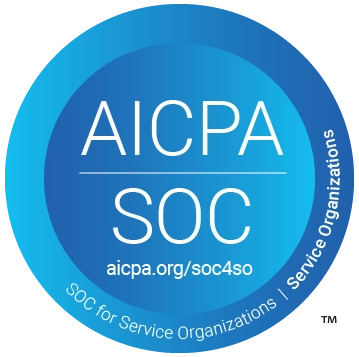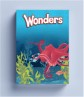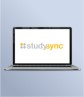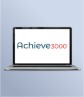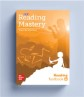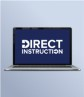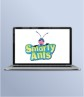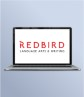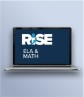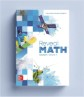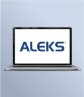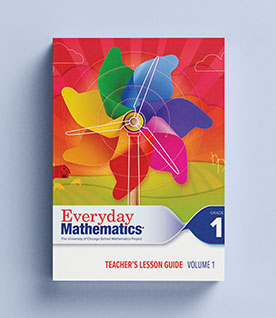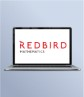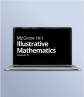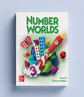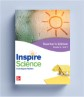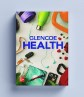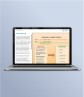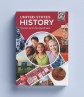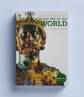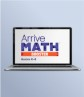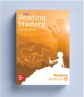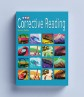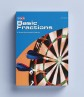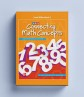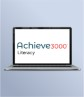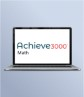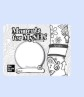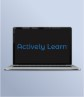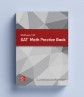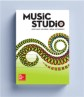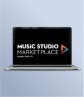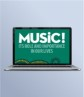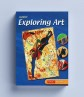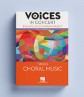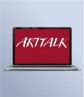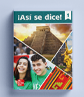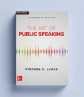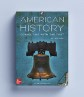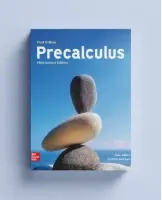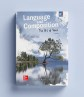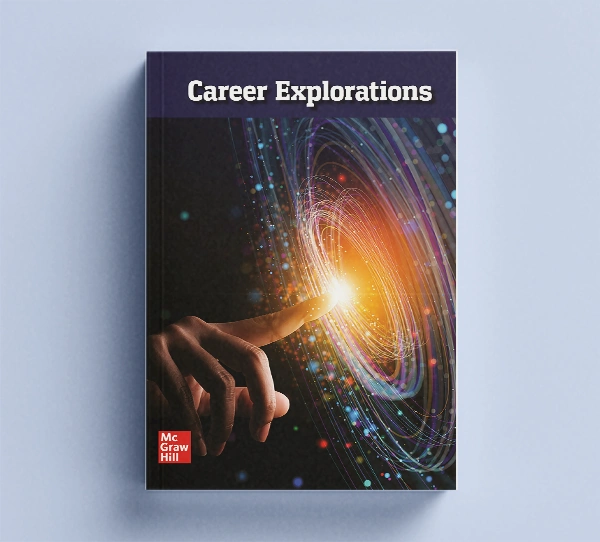## Our Principles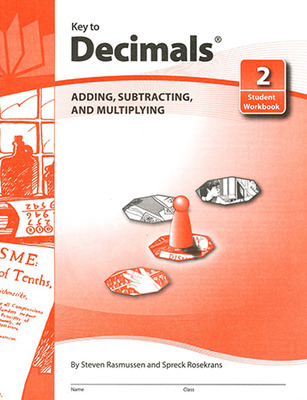# Key to…® Workbooks

Give your students the keys to math-skill mastery! These self-paced, self-guided workbooks, covering topics from fractions and decimals to algebra and geometry, motivate students and build their confidence with bite-size, easy-to-follow lessons.

## Program Details

With Key to...® workbooks, you can customize curriculum for individual learners.

Titles:

• Key to Fractions covers topics from basic concepts to mixed numbers. Handwritten examples provide a non-threatening model, and exercises are structured to ensure student success. Key to Fractions works well for elementary students learning new skills as well as for older students revisiting topics.
• Key to Geometry introduces students to a wide range of geometric discoveries as they do step-by-step constructions. Using only a pencil, compass, and straightedge, students begin by drawing lines, bisecting angles, and reproducing segments. Later they do sophisticated constructions involving over a dozen steps. When they finish, students will have been introduced to 134 geometric terms and will be ready to tackle formal proofs.
• Key to Decimals begins with basic concepts and operations on decimals. Then it covers real-world uses of decimals in pricing, sports, metrics, calculators, and science. Visual models illustrate new concepts, and simple, easy-to-follow instructions let students work on their own or in small groups as they master essential math skills.
• Key to Percents emphasizes mental computation and estimation skills. Then students are taught to solve percent problems using equal fractions and decimal multiplication. Finally, percents are used to solve word problems in a variety of applications. Key to Percents assumes only knowledge of fraction and decimal computation.
• Students using Key to Measurement will enjoy a variety of hands-on experiences related to the English system of measurement. Group projects are included, in addition to numerous individual activities.
• In Key to Metric Measurement students learn how to measure in metric units—the only system used in international commerce and communication, and one becoming used in science and technical fields. Students who learn to use the metric system early will be more comfortable as adults with this form of measurement.
• In Key to Algebra new algebra concepts are explained in simple language, and examples are easy to follow. Word problems relate algebra to familiar situations, helping students understand abstract concepts. Students develop understanding by solving equations and inequalities intuitively before formal solutions are introduced.
• Students begin their study of algebra in Books 1-4 using only integers.
• Books 5-7 introduce rational numbers and expressions.
• Books 8-10 extend coverage to the real number system.
##### Get Help